Studying Gamut Clipping

Introduction

Recently, I was studying a technique called gamut clipping from this blog post. This technique is used for handling out of gamut color and bring them back to a valid range, which helps reducing hue shift and color clipping. From that blog post, it explains the concept clearly, but I was struggling to understand the sample code the author provided. So this blog post will describe what I have learnt when studying the gamut clipping source code. Also, I have written a Shadertoy sample to help me to understand the problem.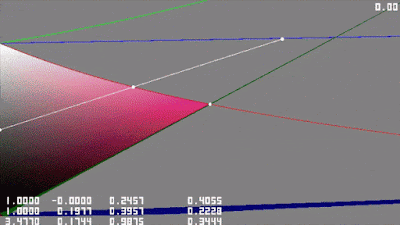Drawing the valid sRGB color with Oklab chroma as horizontal axisOklab lightness as vertical axis with hue value displayed at upper right corner

Overview of the gamut clipping

This section briefly describe the steps to perform gamut clipping, feel free to skip it if you have read the original gamut clipping blog post already. The technique first start to convert the out of gamut color (e.g. those pixels with values >1.0 or <0.0 in sRGB) into the Oklab color space (which can be expressed with lightness, hue and chroma). Then we try to project this out of gamut color along a straight line to the"triangular" gamut boundary (like below picture). To calculate this intersection, we need to find the cusp coordinates at this particular hue slice. The author use curve fitting to approximate cusp coordinates with polynomial equation. With the cusp coordinates calculated, the intersection point can be found with numerical approximation using Halley's method. Finally that intersection point can be converted back to sRGB color space.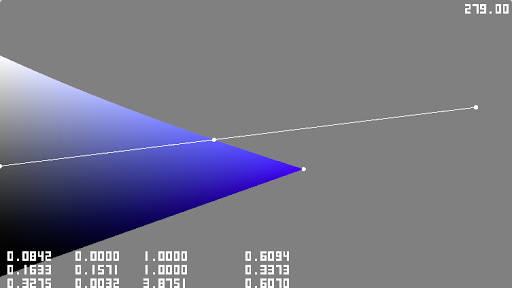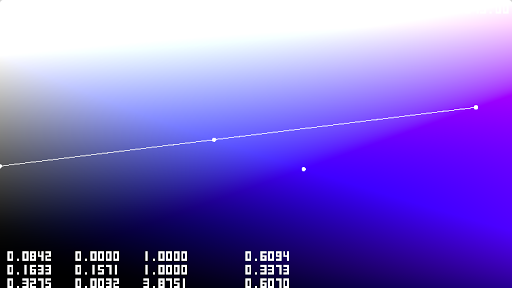Showing an out of gamut color point being projected back to a valid value along a straight line. Displaying all color including those sRGB clipped color, out of gamut color will result in hue shift (e.g. blue hue displayed as purple).

Finding maximum saturation

To approximate the cusp, instead of using the hue value and returning the cusp (chroma, lightness) coordinates directly. The author tries to use the maximum saturation value to find the cusp coordinates. This is the first thing I don't understand when reading the source code. Why saturation is related to the cusp? To understand this, we can start from the definition of saturation first. Both terms chroma and saturation describe colorfulness, but saturation is "somehow normalized" and "not affected" by the brightness. A chroma/lightness/saturation relationship can be defined like this: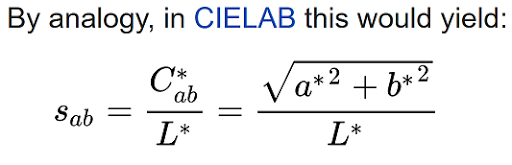Saturation definition for CIELAB space from Wiki,we use the same definition for Oklab space too.
Reading the author's Colab source code, he tried to approximate the max saturation with a polynomial function using a=cos(hue) and b=sin(hue):

Then these (saturation, hue) values will be converted to linear sRGB space and minimize it for R=0, G=0 and B=0, which obtained 3 polynomial equations to approximate the maximum saturation.

But why we can obtain maximum saturation when either R / G / B=0? To have a rough understanding of it, I decided to open a color picker with HSL slider to "simulate" how the RGB values changes: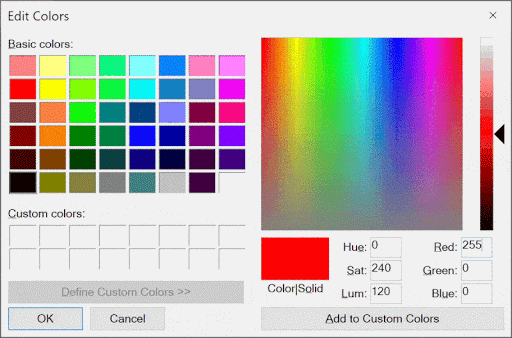A color picker with maximum saturation and changing hue slowly.There will always be a 0 in the RGB value.

First I choose the most saturated red color (255, 0, 0) in sRGB, which yield a HSL value (0, 240, 120). Then I change the hue value slowly to observe how the RGB value changes. From the above animated gif, we can see that there will always be a 0 in either R or G or B channel. So I guess the author is using this property and the Oklab space has similar property.

To further understand how the saturation looks like for all hue slices in Oklab, I plotted the saturation value, seems like it always have the largest value at the lower "triangle" edge:

We can also draw the line for those pixels with R/G/B value = 0:

The lower "triangle" edge is actually the "clipping" lines when R=0 or G=0 or B=0 (and switching between these 3 lines)! That's why the author tried to use 3 different polynomials to approximate the max saturation. Then the next problem is how to pick one of the three approximated polynomials. From the above animated gif, we know that the "clipping line" changes when sRGB values = (1, 0, 0), (0, 1,0) or (0, 0, 1). We can see this from the Colab sample code which find the r_dir/g_dir/b_dir, and use this to pick one of the three approximated polynomials.

The r_dir/g_dir/b_dir is the "half vector" between the "opposite hue" like the below figure. And this direction vector can be used with dot product to check whether a hue value (in form of cos(hue), sin(hue)) is lying within which r_h/g_h/b_h range.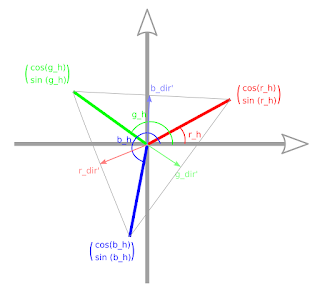Visualizing the r/g/b_dir' vector (before multiplying the scalar constant for fast dot product check).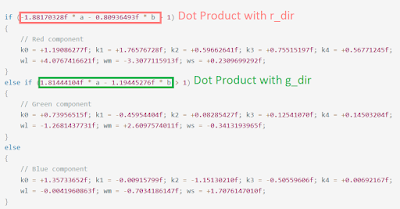The formula for checking which hue range the a, b hue value is in, which can be derived from dot product.

Another problem I don't understand is: when the author tried to minimize the RGB value to 0, he raised the error value to the power 10 in the e_R(x)/e_G(x)/e_B(x) function.

When I tried to throw random values to the initial guess value in scipy.optimize.minimize() (e.g. use all 0 or 1 as initial guess), the resulting approximated curve is not that good...

However when I changed the pow(x, 10) to abs(x), using random initial guess values can have a more predictable result (though the error is not as small as the author's approximation). May be I will use abs(x) instead to optimize for different color spaces in the future.

Finding cusp from maximum saturation

With the above polynomial approximation, we can find the "lower clipping line" of the gamut "triangle" (i.e. with either R/G/B value = 0). The cusp must be on this "clipping line". We only need to identify the lightness value at the cusp. Looking at the author's find_cusp() function, it seems like the cusp will have R or G or B value equals to 1:

To have a better understanding, we can repeat the above color picker "experiment", when changing the hue value at maximum saturation, beside there always having a 0 value in R/G/B, there will always having a 255 value in R/G/B too!The same color picker gif as above,pay attention to the RGB values,in addition to always having a 0 value,there is a 255 value too.

Plotting the line when R/G/B value = 1 for all hue slices in Oklab space:

When R/G/B value = 1, it is the upper clipping line of the "gamut triangle"! So the cusp is the intersection between lower clipping line with R/G/B=0 and the upper clipping line with R/G/B=1.

So, to obtain the maximum lightness and maximum saturation, we can scale the lightness so that when converted back to sRGB, one of the RGB value will = 1 (taking cubic root is needed because of the Oklab color space LMS definition).

Finding gamut intersection

With the gamut cusp coordinates, target project coordinates and out of gamut coordinates, we can find the intersection coordinates at the gamut boundary during projection. First we need to check whether the intersection is in the upper half or the lower half of the valid range gamut "triangle". This can be determined by below formula which can be derived from checking whether the projection line is on the left/right side to the cusp line using cross product.

If in the lower half, it is just a simple line-line intersection. If in the upper half, we first approximate the intersection with line-line intersection, and then refine the answer with Halley's method. Since we know the upper clipping line is R/G/B=1, we can see the author is using this property when using Halley's method in the following code snippet:

and the author use all 3 clipping curves and take the minimum value to select the closest curves that clip the gamut.

If small error is acceptable, we can use 1 clipping curve instead of 3. The upper clipping curve changes happens roughly at RGB value=(0, 1, 1), (1, 0, 1) and (1, 1, 0). We can use the same method as picking the R/G/B=0 curve during maximum saturation calculation which relies on the r_dir, g_dir, b_dir. We can compute the c_dir, m_dir and y_dir (which correspond to the cyan, magenta and yellow direction). Those coefficients can be found in my Shadertoy source code. Because the upper clipping curve is not straight line, we may need 2 clipping lines to compute the correct answer for some hue values:Need 2 upper clipping lines for this hue slice

Conclusion

In this post, I have described the process of learning the gamut clipping technique. With the help of the Shadertoy sample, we can see that the gamut boundary is the line with R/G/B=0 and R/G/B=1. And the gamut cusp is the intersection between R/G/B=0 line and R/G/B=1 line. But I still have some questions left in my mind like: Is it meaningful to use negative chroma values? Is the gamut clipping works well in pratice, or need a gamut compression method? May be I will implement gamut clipping in my toy path tracer to see how it looks like.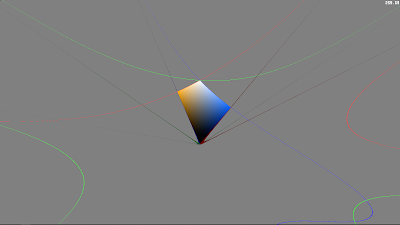Zooming out the graph, do the negative values (e.g. -ve chroma, -ve lightness) have meaning?The G=1 clipping line "wrap around" to negative lightness, does it have meaning too?

Reference